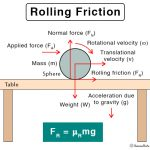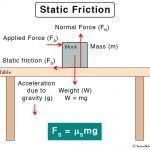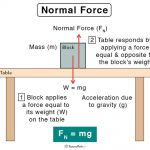Home / Physics / Plane Mirror

# Plane Mirror

## Definition: What is Plane Mirror?

A plane mirror is a mirror that has a flat reflecting surface without any inward or outward curve. A ray of light falling on a plane mirror is reflected at the same angle as the angle of incidence. As a result, the image formed by the mirror is sharp and undistorted. This image is not real but virtual because the image formed behind the mirror by extending the diverging reflected rays backward.

## Properties and Characteristics of a Plane Mirror

1. The image is virtual and upright.
2. The image is of the same size as that of the object, i.e., magnification = 1.
3. The distance of the object from the mirror (object distance, o) is the same as that of the image from the mirror (image distance, i). Hence, the plane mirror equation is: i = o
4. The image is laterally inverted, i.e., if the left hand is raised, it appears, as though the right hand has been raised on the image. This phenomenon is known as lateral inversion.

## Laws of Reflection

There are two laws of reflection that are applicable to a plane mirror.

1. The angle of incidence is equal to the angle of reflection.
2. The incident ray, the reflected ray, and normal lie on the same plane.

## Differences Between Plane Mirror and Curved Mirror

### Plane Mirror vs. Curved Mirror

Plane Mirror Curved Mirror
Is flat Is curved
Produces virtual image Produces both real and virtual image
Has an infinite focal length and radius of curvature Has a finite focal length and radius of curvature
Has an equation given by i = o Has an equation given by 1/i + 1/o = 1/R

## Uses and Applications of Plane Mirror

• At home in daily life, especially in the bathroom and bedroom and as a wall mirror
• As a makeup and shaving mirror
• In homemade periscope and kaleidoscope used by children
• In SLR camera and overhead projector
• As optical levers in scientific instruments

## FAQs

Q.1. What is the focal length of a plane mirror?

Ans. The focal length of a plane mirror is infinity.

Q.2. Can a plane mirror form multiple images?

Ans. Yes. If two or more plane mirrors are placed at a certain angle, then multiple images can be formed.

Q.3. How many images are formed by two parallel plane mirrors?

Ans. Two parallel plane mirrors can form an infinite number of images.

Q.4. Why are ordinary plane mirrors coated on the back?

Ans. Ordinary plane mirrors are coated at the back so that the incident ray is reflected from the back surface instead of the front surface. The front surface can be damaged easily due to scratches and watermarks.

Q.5. Can a plane mirror form an inverted image?

Ans.No. A plane mirror cannot form an inverted image.

Article was last reviewed on Tuesday, July 7, 2020

### Related articlesRolling FrictionSliding FrictionStatic FrictionNormal Force